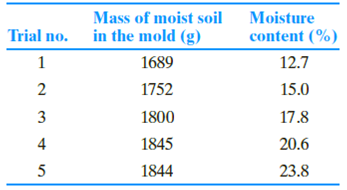Chapter 6, Problem 6.8PPrinciples of Geotechnical Enginee...

9th Edition
Braja M. Das + 1 other
ISBN: 9781305970939

Solutions

Chapter
SectionPrinciples of Geotechnical Enginee...

9th Edition
Braja M. Das + 1 other
ISBN: 9781305970939
Textbook Problem

A standard Proctor test was conducted on a silty clay soil collected from a proposed construction site. The results are shown in the following table.a. Determine the maximum dry density (kg/m3) of compaction and the optimum moisture content. Given: Mold volume = 943.3 cm3.b. If specification calls for 99% relative compaction in the field, what would be the field dry density and the range of acceptable moisture content?

(a)

To determine

The maximum dry density of compaction and the optimum moisture content.

Explanation

Given information:

The volume of mold (V) is 943.3cm3.

Calculation:

Calculate the density of soil for trial 1 using the relation.

ρ=MV

Here, M is the mass of soil in the mold for trial 1.

Substitute 1,689 g for M and 943.3cm3 for V.

ρ=1,689943.3=1.7905g/cm3×1kg1,000g×(100cm1m)3=1,790.5kg/m3

Similarly, calculate the density of soil for remaining trials.

Calculate the dry density of soil for trial 1 using the relation.

ρd=ρ1+w100

Here, w is the moisture content for trial 1.

Substitute 1,790.5kg/m3 for ρ and 12.7 % for w.

ρd=1,790.51+12.7100=1,588.7kg/m3

Similarly, calculate the dry unit weight of soil mass for remaining trials

(b)

To determine

The field dry density and range of acceptable moisture content.

Still sussing out bartleby?

Check out a sample textbook solution.

See a sample solution

The Solution to Your Study Problems

Bartleby provides explanations to thousands of textbook problems written by our experts, many with advanced degrees!

Get Started